# Convert dataframe rows and columns to vector in R

In this article, we are going to convert a dataframe column to a vector and a dataframe row to a vector in the R Programming Language.

### Convert dataframe columns into vectors

We are taking a column in the data frame and passing it into another variable by using the selection method. The selection method can be defined as choosing a column from a data frame using the ” [[]]” operator.

Steps –

• Create data frame
• Select column to be converted
• Assign it to a variable
• Display data frame so generated.

Syntax:

dataframe[[‘column’]]

Example:

## R

 `# create vectors ` `id=``c``(7058,7084,7098) ` `name=``c``(``'sravan'``,``'karthik'``,``'nikhil'``) ` ` `  `# passing into dataframe ` `data=``data.frame``(id,name)  ` `print``(data) ` ` `  `# convert id column into a vector ` `column_data=data[[``'id'``]] ` `print``(column_data) ` ` `  `# convert name column into a vector ` `column_data1=data[[``'name'``]] ` `print``(column_data1)`

Output: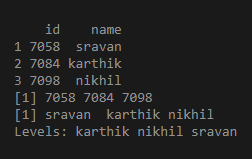### Convert data frame row to a vector

We can convert every row or entire dataframe by using a method called as.vector()

Approach

• Create dataframe
• Select row to be converted
• Pass it to the function
• Display result

Syntax:

as.vector(t(dataframe_name))

Where t is the transpose of the dataframe. If t is not specified, the output is both rows and column names. If it is specified output is only rows.

Example: without t specification.

## R

 `# create vectors ` `id=``c``(7058,7084,7098) ` `name=``c``(``'sravan'``,``'karthik'``,``'nikhil'``) ` ` `  `# passing into dataframe ` `data=``data.frame``(id,name)  ` `print``(data) ` `print``(``"-----------"``) ` ` `  `# converting 1 st row to a vector ` `as.vector``((data[1,])) ` `print``(``"-----------"``) ` ` `  `# converting 2nd row to a vector ` `as.vector``((data[2,])) ` `print``(``"-----------"``) ` ` `  `# converting 3 rd row to a vector ` `as.vector``((data[3,])) ` `print``(``"-----------"``) `

Output: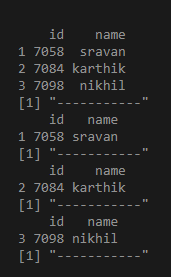Example: Using t

## R

 `# create vectors ` `id=``c``(7058,7084,7098) ` `name=``c``(``'sravan'``,``'karthik'``,``'nikhil'``) ` ` `  `# passing into dataframe ` `data=``data.frame``(id,name)  ` `print``(data) ` `print``(``"-----------"``) ` ` `  `# converting 1 st row to a vector ` `as.vector``(``t``(data[1,])) ` `print``(``"-----------"``) ` ` `  `# converting 2nd row to a vector ` `as.vector``(``t``(data[2,])) ` `print``(``"-----------"``) ` ` `  `# converting 3 rd row to a vector ` `as.vector``(``t``(data[3,])) ` `print``(``"-----------"``) `

Output: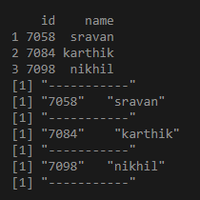Example:

## R

 `# create vectors ` `id=``c``(7058,7084,7098) ` `name=``c``(``'sravan'``,``'karthik'``,``'nikhil'``) ` ` `  `# passing into dataframe ` `data=``data.frame``(id,name)  ` `print``(data) ` `print``(``"-----------"``) ` ` `  `# converting all dataframe  to a vector ` `as.vector``(``t``(data)) `

Output: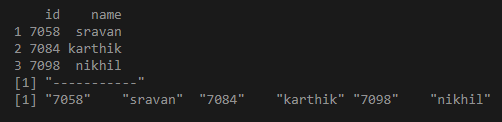Example 2 :

## R

 `# create vectors ` `id=``c``(7058,7084,7098) ` `address=``c``(``'guntur'``,``'hyd'``,``'kothapeta'``) ` `name=``c``(``'sravan'``,``'karthik'``,``'nikhil'``) ` ` `  `# passing into dataframe ` `data=``data.frame``(id,address,name)  ` `print``(data) ` `print``(``"-----dataframe row to a vector------"``) ` ` `  `# converting dataframe to a vector ` `as.vector``(``t``(data)) ` ` `  `print``(``"-----dataframe column to a vector------"``) ` ` `  `# converting dataframe 1 st  column to a vector ` `data1=data[[``'id'``]] ` `print``(data1) ` ` `  `# converting dataframe 2 nd  column to a vector ` `data1=data[[``'address'``]] ` `print``(data1) ` ` `  `# converting dataframe 3 rd  column to a vector ` `data1=data[[``'name'``]] ` `print``(data1) `

Output: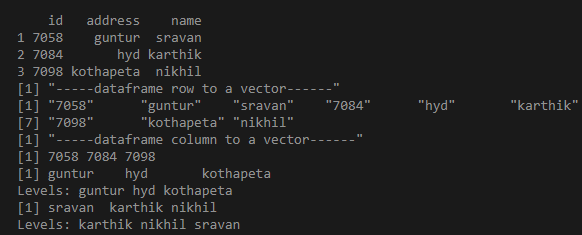Whether you're preparing for your first job interview or aiming to upskill in this ever-evolving tech landscape, GeeksforGeeks Courses are your key to success. We provide top-quality content at affordable prices, all geared towards accelerating your growth in a time-bound manner. Join the millions we've already empowered, and we're here to do the same for you. Don't miss out - check it out now!

Previous
Next